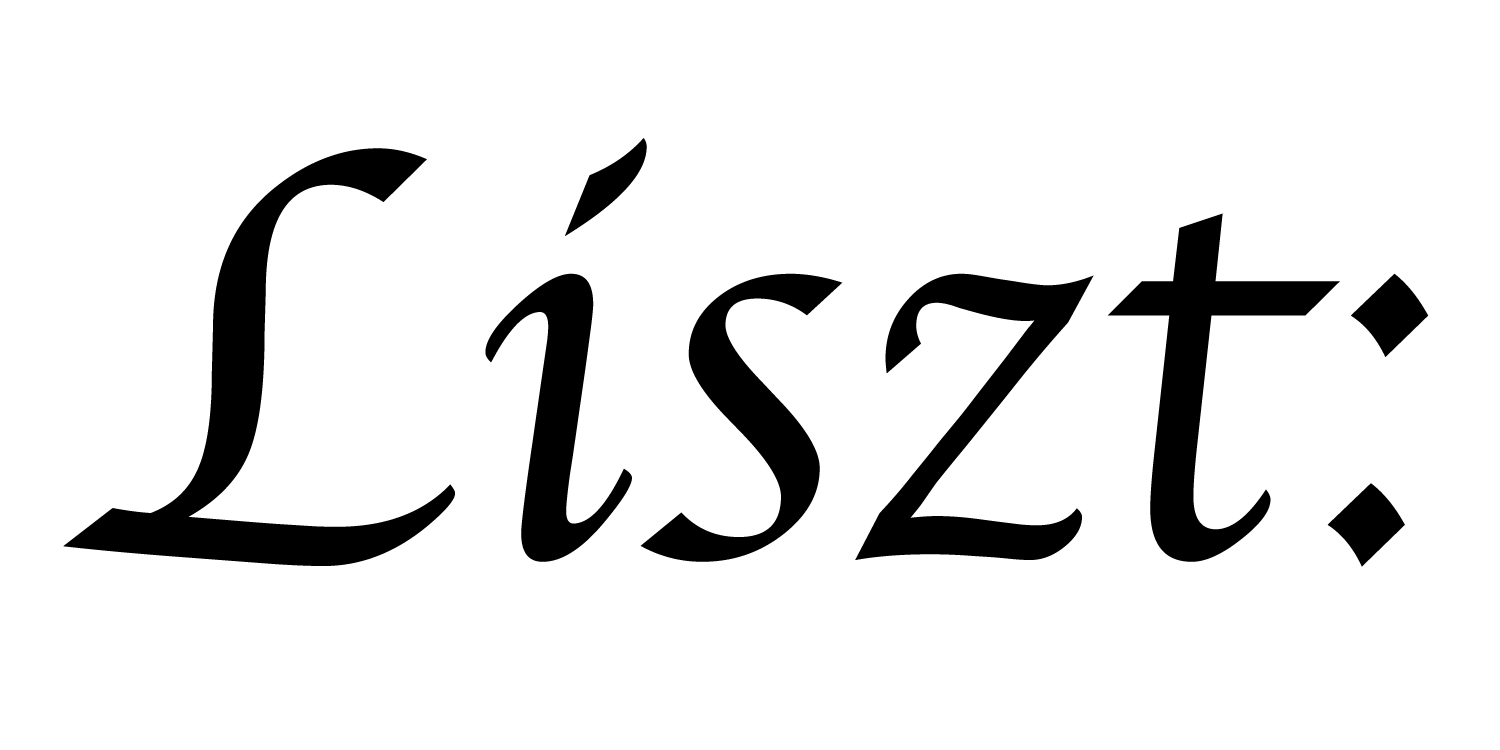# 11: Calling C-code

In this tutorial, we’ll repeat the tile-swapping variant of the heat-diffusion simulation from tutorial 10, but instead of implementing the tile swap computation in Terra, we’ll use traditional C-code.

That C-code is in file `11___c_code.c` and `11___c_header.h`. It needs to be separately compiled into a shared object/library. We’ve included some sample compilation commands in a makefile in this directory.

## C Code

``````#include "11___c_header.h"
#include "ebb/lib/dld.h"
``````

In order to get access to the struct layout, we include the C-header file description of DLDs from the Ebb standard library.

Notice that this version of the `tile_shuffle()` function also reads the dimensions of the 2d grid, rather than assuming it will be 40x40.

``````void tile_shuffle( void * dld_ptr ) {
DLD *dld        = (DLD*)(dld_ptr);
int xstride     = (int)(dld->dim_stride);
int ystride     = (int)(dld->dim_stride);
int xdim        = (int)(dld->dim_size);
int ydim        = (int)(dld->dim_size);
int halfx       = xdim / 2;
int halfy       = ydim / 2;

for (int y=0; y<halfy; y++) {
for (int x=0; x<halfx; x++) {
int t1_idx  =         x * xstride   +         y * ystride;
int t2_idx  = (x+halfx) * xstride   + (y+halfy) * ystride;

double temp   = t_ptr[t1_idx];
t_ptr[t1_idx] = t_ptr[t2_idx];
t_ptr[t2_idx] = temp;
}
}
}
``````

## Ebb Code

``````import 'ebb'
local L = require 'ebblib'

local GridLib   = require 'ebb.domains.grid'

local DLD       = require 'ebb.lib.dld'
local vdb       = require 'ebb.lib.vdb'

local N = 40

local grid = GridLib.NewGrid2d {
size   = {N, N},
origin = {0, 0},
width  = {N, N},
periodic_boundary = {true,true},
}

local timestep    = L.Constant(L.double, 0.45)
local conduction  = L.Constant(L.double, 1.0)

local function init_temperature(x_idx, y_idx)
if x_idx == 4 and y_idx == 6 then return 400
else return 0 end
end

local ebb visualize ( c : grid.cells )
vdb.color({ 0.5 * c.t + 0.5, 0.5-c.t, 0.5-c.t })
var p2 = c.center
vdb.point({ p2, p2, 0 })
end

local ebb update_temperature ( c : grid.cells )
var avg   = (1.0/4.0) * ( c(1,0).t + c(-1,0).t + c(0,1).t + c(0,-1).t )
var diff  = avg - c.t
c.new_t   = c.t + timestep * conduction * diff
end
``````

The bulk of this simulation is exactly identical to the previous tutorial 10 on DLDs.

``````local PN    = require 'ebb.lib.pathname'
local here  = tostring(PN.scriptdir():abspath())

{'-I'..here,
'-I'..here..'/../../include'})
``````

Here we use the Terra API calls `linklibrary()` and `includecstring()` to first dynamically link the shared object into the process and then import the header file’s interface. To do so, we need to make sure Terra knows where to look for the shared object and for all the header files being included. Rather than hard-code fragile paths, we again use the pathname library to dynamically determine the correct filesystem paths based on the location of this script file.

Once these calls have run, the C_Import table will contain a Terra/C function `tile_shuffle()`, that we can call to invoke the C code. For more information on how this whole process works, take a look at the Terra documentation.

``````for i=1,360 do
grid.cells:foreach(update_temperature)
grid.cells:Swap('t', 'new_t')

if i % 200 == 199 then
local t_dld = grid.cells.t:GetDLD()

assert( t_dld.version == 1 and t_dld.version == 0 )
assert( t_dld.base_type      == DLD.DOUBLE )
assert( t_dld.location       == DLD.CPU )
assert( t_dld.type_stride    == 8 )
assert( t_dld.type_dims == 1 and t_dld.type_dims == 1 )
assert( t_dld.dim_size == 1 )

C_Import.tile_shuffle( t_dld:toTerra() )
end

vdb.vbegin()
vdb.frame()
grid.cells:foreach(visualize)
vdb.vend()

if i % 10 == 0 then print( 'iteration #'..tostring(i) ) end
end
``````

The simulation loop then, is nearly identical to the simulation loop from tutorial 10.

a part of the Liszt project and PSAAP II center at Stanford University